Courses
Courses for Kids
Free study material
Offline Centres
MoreLast updated date: 04th Dec 2023
Total views: 281.7k
Views today: 3.81k

# Match the following?(i) Cone(a)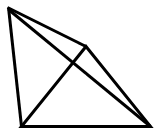(ii) Sphere(b)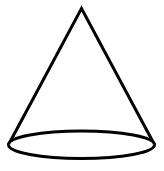(iii) Cube(c)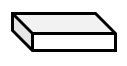(iv) Pyramid(d)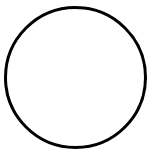(v) Cylinder(e)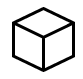(vi) Cuboid(f)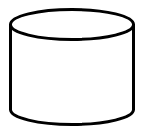Verified
281.7k+ views
Hint: First we will understand about each of the shapes given in the options by considering their figures and we will know about some of their properties. For cube: - match it with the figure that has 6 flat surfaces with each being the square. For cuboid: - match it with the figure that has 6 surfaces containing three types of rectangles, and so on.

Here we have been provided with the options containing the names of figures in column 1 and in column 2 we have the diagram of the figures. We are asked to match them correctly. Let us understand all the shapes given in the options one by one.
(i) ConeA cone is a 3 – D figure that has a flat circular surface known as the base of the cone and a curved surface that can be obtained by folding a semicircle along its diameter in a suitable manner.
(ii) SphereA sphere is a round 3 – D figure as shown in the above figure. Basketballs, footballs etc. are some examples of spheres in real world.
(iii) Cube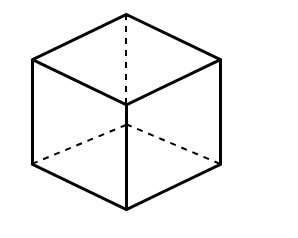A cube a 3 – D figure that has 6 flat surfaces, 12 edges and 8 vertices. The lengths of all the arms are equal and therefore we can say that it is made up of 6 squares of equal sides.
(iv) Pyramid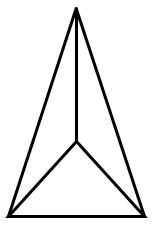Pyramid is shown in the above figure and it is of many types like: - triangular pyramid, square pyramid, rectangular pyramid, pentagonal pyramid etc. In the above figure we have a triangular because in the option we see that the base of the pyramid is a triangle.
(v) CylinderA Cylinder is a 3 – D object which has two circular flat surfaces at the ends and one curved surface that can be obtained by folding a rectangle either along its length or breadth.
(vi) Cuboid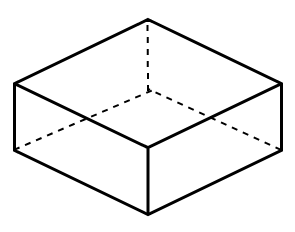A cuboid has also 6 flat surfaces, 12 edges and 8 vertices but the difference between a cube and a cuboid is that in cuboid we have the dimensions of its length, breadth and height all different from each other.
Hence, on reading about the above shapes we can match the names of shapes with their correct diagram as follows: -
 (i) Cone (b)(ii) Sphere (d)(iii) Cube (e)(iv) Pyramid (a)(v) Cylinder (f)(vi) Cuboid (c)Note: Note that there are many other 3 – D shapes also about which we must know like prism, frustum of a cone, tetrahedron etc. There are many types of prisms like there are for the pyramid. The formula for the volume, curved surface area and total surface area of all the figures mentioned in the question must be remembered as they will be used in further topics of mathematics.